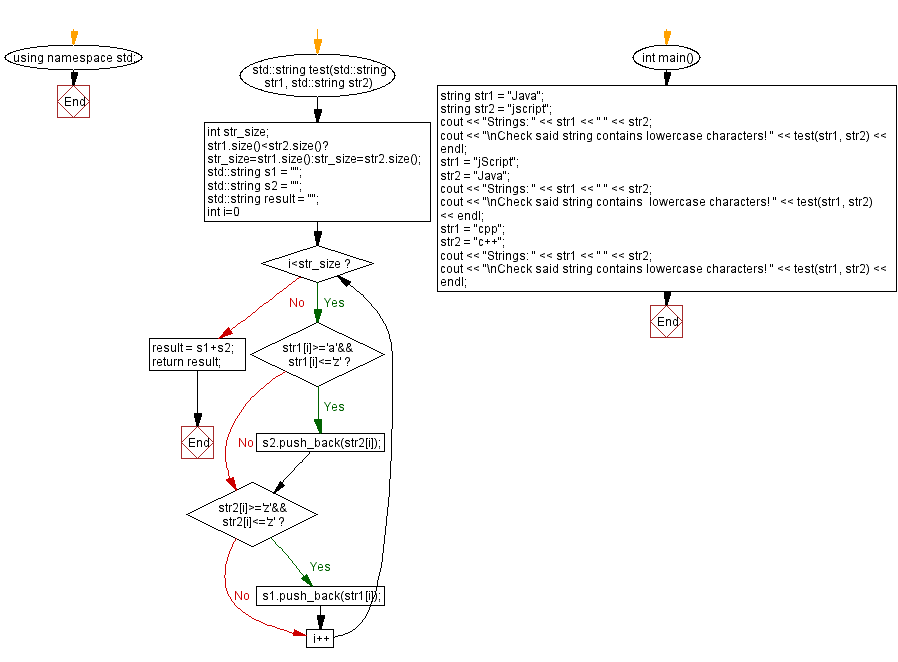﻿ C++ - Select lowercase characters from the other string

# C++ String Exercises: Select lowercase characters from the other string

## C++ String: Exercise-40 with Solution

For two given strings, str1 and str2, write a C++ program to select only the characters that are lowercase in the other string at the same position. Return characters as a single string.

Test Data:
("Java", "jscript") -> "scr"
("jScript", "Java") -> "Jva"
("cpp", "c++") -> "c++"

Sample Solution:

C++ Code:

``````#include <bits/stdc++.h>

using namespace std;

std::string test(std::string str1, std::string str2) {
int str_size;
str1.size()<str2.size()? str_size=str1.size():str_size=str2.size();
std::string s1 = "";
std::string s2 = "";
std::string result = "";
for (int i=0;i<str_size;i++)
{
if (str1[i]>='a'&&str1[i]<='z')
s2.push_back(str2[i]);
if (str2[i]>='z'&&str2[i]<='z')
s1.push_back(str1[i]);
}
result = s1+s2;
return result;
}

int main()
{
string str1 = "Java";
string str2 = "jscript";
cout << "Strings: " << str1 << " " << str2;
cout << "\nCheck said string contains lowercase characters! " << test(str1, str2) << endl;
str1 = "jScript";
str2 = "Java";
cout << "Strings: " << str1 << " " << str2;
cout << "\nCheck said string contains lowercase characters! " << test(str1, str2) << endl;
str1 = "cpp";
str2 = "c++";
cout << "Strings: " << str1 << " " << str2;
cout << "\nCheck said string contains lowercase characters! " << test(str1, str2) << endl;

}
``````

Sample Output:

```Strings: Java jscript
Check said string contains lowercase characters! scr
Strings: jScript Java
Check said string contains lowercase characters! Jva
Strings: cpp c++
Check said string contains lowercase characters! c++
```

Flowchart:C++ Code Editor: